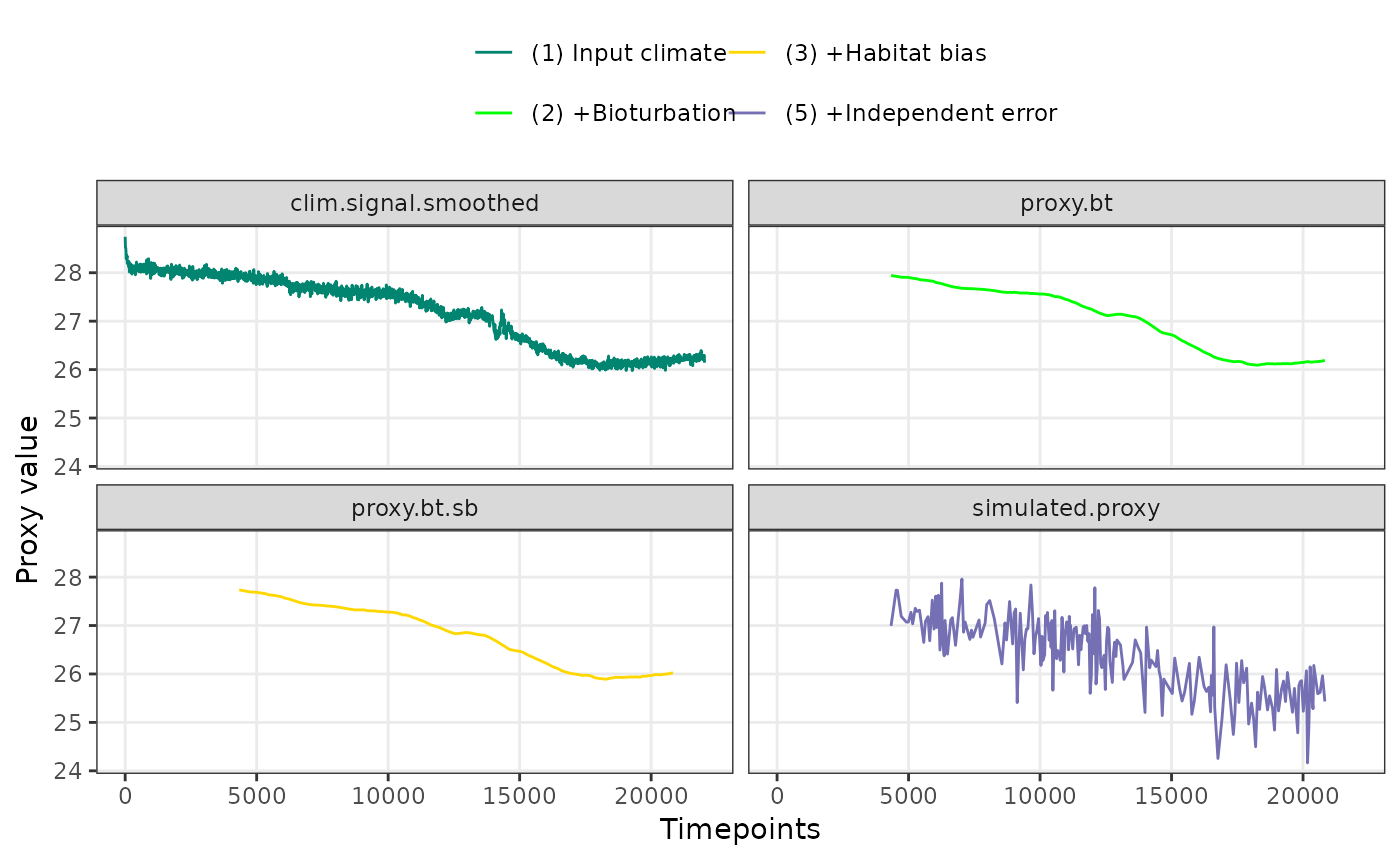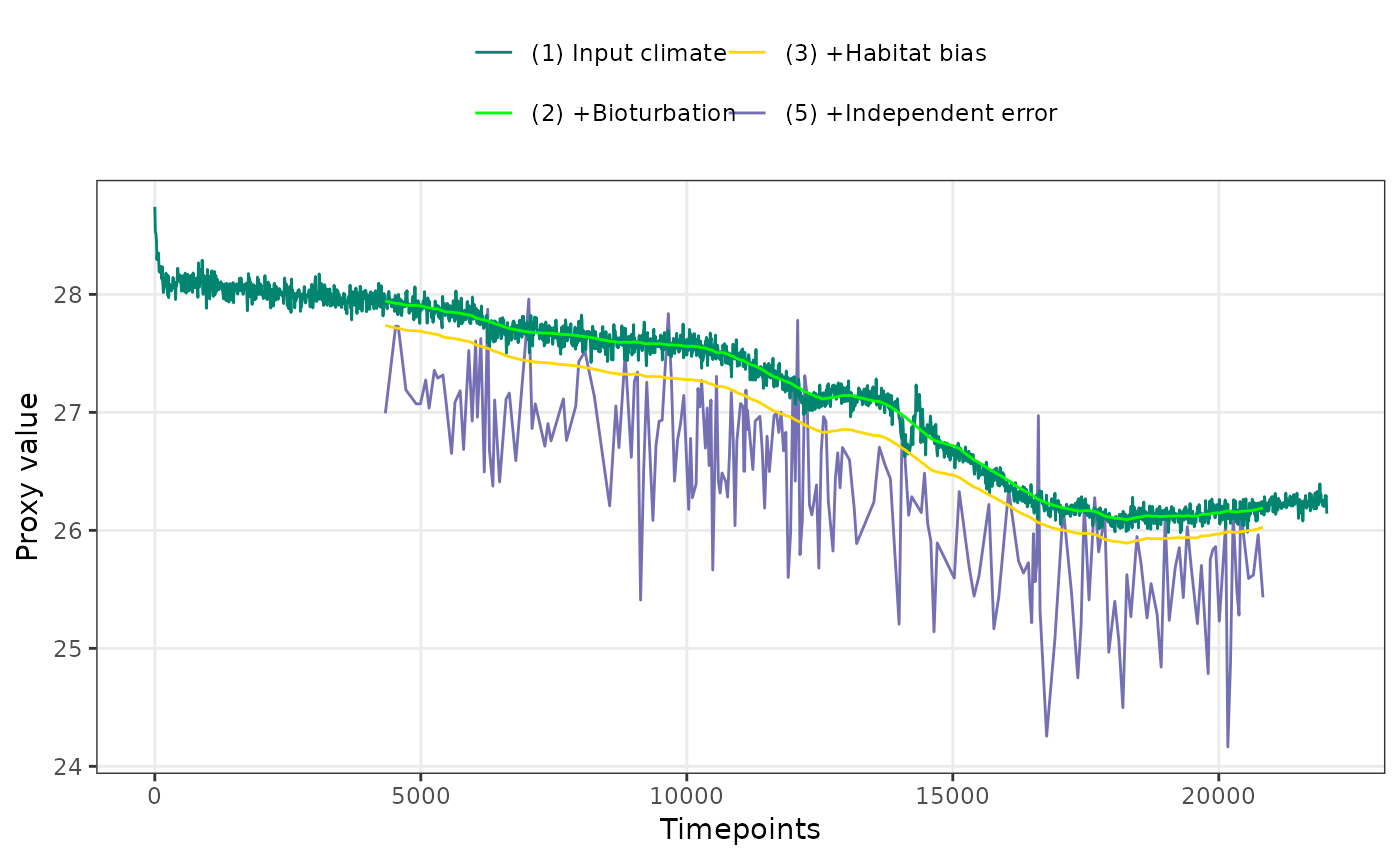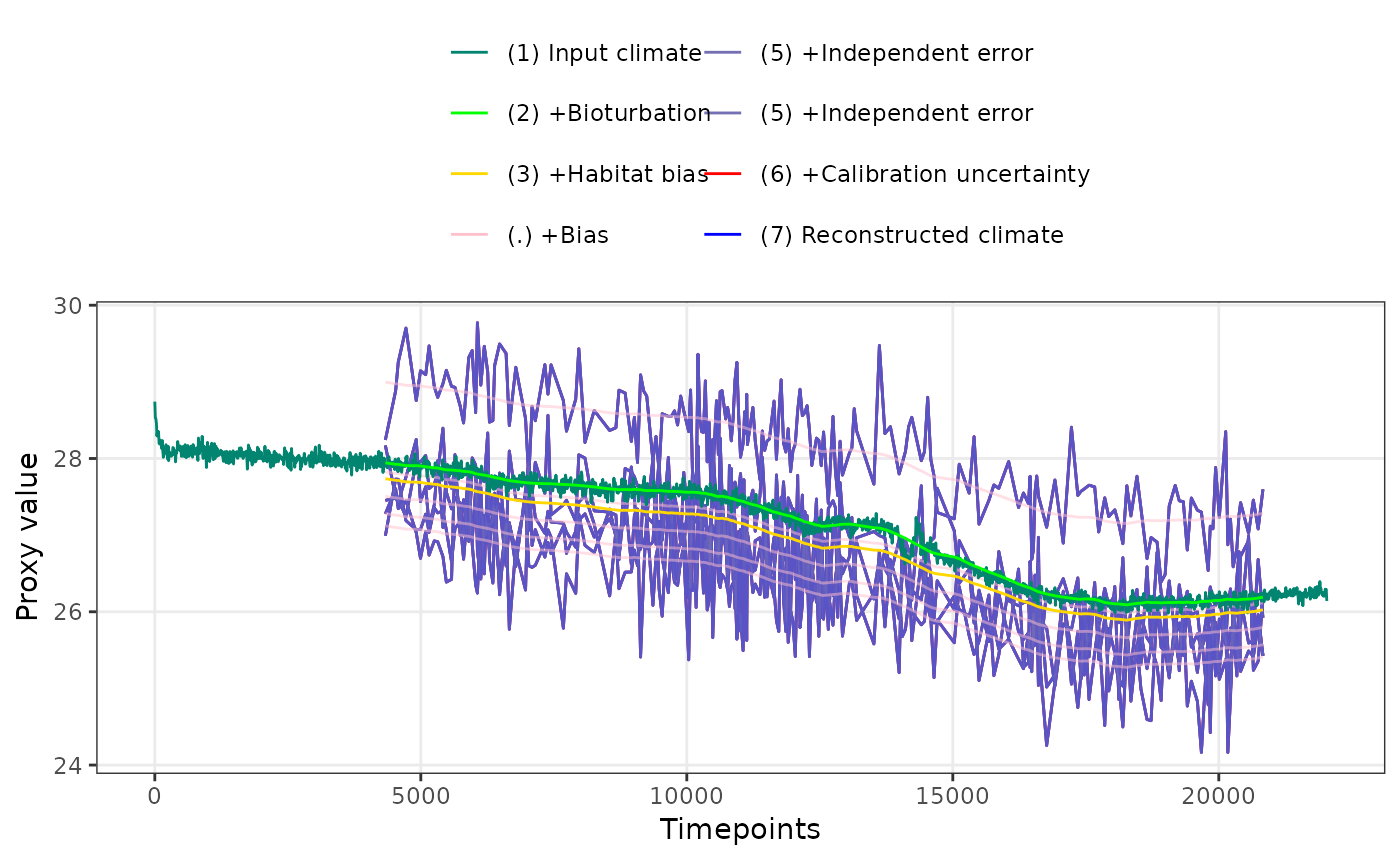Plot forward modelled sedimentary proxies

## Usage

PlotPFMs(
PFMs,
stage.order = c("var", "seq"),
plot.stages = c("default"),
max.replicates = 5,
colr.palette = "default",
alpha.palette = "default",
levl.labels = "default"
)

## Arguments

PFMs

A dataframe of forward modelled proxies

stage.order

Controls the order in which proxy stages are plotted, either sequentially, "seq", or in order of variance, "var". Defaults to var.

plot.stages

Proxy stages to be plotted, "default", "all", or a custom character vector

max.replicates

Maximum number of replicates to plot at once

colr.palette

Colours for the proxy stages

alpha.palette

Alpha levels for the proxy stages

levl.labels

Labels for the proxy stages

## Value

a ggplot object of class "gg" "ggplot"

## Examples

library(ggplot2)
set.seed(26052017)
clim.in <- ts(N41.t21k.climate[nrow(N41.t21k.climate):1,] - 273.15)

PFM <- ClimToProxyClim(clim.signal = clim.in,
timepoints = round(N41.proxy$Published.age), calibration.type = "identity", habitat.weights = N41.G.ruber.seasonality, sed.acc.rate = N41.proxy$Sed.acc.rate.cm.ka,
sigma.meas = 0.45,
sigma.ind = 0,
n.samples = Inf,
plot.sig.res = 10, meas.bias = 1,
n.replicates = 10)

PlotPFMs(PFM$everything, max.replicates = 1, stage.order = "seq") + facet_wrap(~stage) #> Joining with by = join_by(stage, scale) #> Scale for alpha is already present. #> Adding another scale for alpha, which will replace the existing scale.PlotPFMs(PFM$everything, max.replicates = 1, stage.order = "var")
#> Joining with by = join_by(stage, scale)
#> Scale for alpha is already present.
#> Adding another scale for alpha, which will replace the existing scale.PlotPFMs(PFM\$everything, stage.order = "var", plot.stages = "all")
#> Joining with by = join_by(stage, scale)
#> Scale for alpha is already present.
#> Adding another scale for alpha, which will replace the existing scale.